## 4.14.4. Subarray Datatype Constructor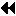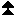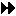Up: New Datatype Manipulation Functions Next: Distributed Array Datatype Constructor Previous: True Extent of Datatypes

MPI_TYPE_CREATE_SUBARRAY(ndims, array_of_sizes, array_of_subsizes, array_of_starts, order, oldtype, newtype)
[ IN ndims] number of array dimensions (positive integer)
[ IN array_of_sizes] number of elements of type oldtype in each dimension of the full array (array of positive integers)
[ IN array_of_subsizes] number of elements of type oldtype in each dimension of the subarray (array of positive integers)
[ IN array_of_starts] starting coordinates of the subarray in each dimension (array of nonnegative integers)
[ IN order] array storage order flag (state)
[ IN oldtype] array element datatype (handle)
[ OUT newtype] new datatype (handle)

int MPI_Type_create_subarray(int ndims, int array_of_sizes[], int array_of_subsizes[], int array_of_starts[], int order, MPI_Datatype oldtype, MPI_Datatype *newtype)
MPI_TYPE_CREATE_SUBARRAY(NDIMS, ARRAY_OF_SIZES, ARRAY_OF_SUBSIZES, ARRAY_OF_STARTS, ORDER, OLDTYPE, NEWTYPE, IERROR)
INTEGER NDIMS, ARRAY_OF_SIZES(*), ARRAY_OF_SUBSIZES(*), ARRAY_OF_STARTS(*), ORDER, OLDTYPE, NEWTYPE, IERROR
MPI::Datatype MPI::Datatype::Create_subarray(int ndims, const int array_of_sizes[], const int array_of_subsizes[], const int array_of_starts[], int order) const

The subarray type constructor creates an MPI datatype describing an n-dimensional subarray of an n-dimensional array. The subarray may be situated anywhere within the full array, and may be of any nonzero size up to the size of the larger array as long as it is confined within this array. This type constructor facilitates creating filetypes to access arrays distributed in blocks among processes to a single file that contains the global array.

This type constructor can handle arrays with an arbitrary number of dimensions and works for both C and Fortran ordered matrices (i.e., row-major or column-major). Note that a C program may use Fortran order and a Fortran program may use C order.

The ndims parameter specifies the number of dimensions in the full data array and gives the number of elements in array_of_sizes, array_of_subsizes, and array_of_starts.

The number of elements of type oldtype in each dimension of the n-dimensional array and the requested subarray are specified by array_of_sizes and array_of_subsizes, respectively. For any dimension i, it is erroneous to specify array_of_subsizes[i] < 1 or array_of_subsizes[i] > array_of_sizes[i].

The array_of_starts contains the starting coordinates of each dimension of the subarray. Arrays are assumed to be indexed starting from zero. For any dimension i, it is erroneous to specify array_of_starts[i] < 0 or array_of_starts[i] > ( array_of_sizes[i] - array_of_subsizes[i]).

In a Fortran program with arrays indexed starting from 1, if the starting coordinate of a particular dimension of the subarray is n, then the entry in array_of_starts for that dimension is n-1. ( End of advice to users.)
The order argument specifies the storage order for the subarray as well as the full array. It must be set to one of the following:

{ MPI_ORDER_C}
The ordering used by C arrays, (i.e., row-major order)
{ MPI_ORDER_FORTRAN}
The ordering used by Fortran arrays, (i.e., column-major order)

A ndims-dimensional subarray ( newtype) with no extra padding can be defined by the function Subarray() as follows: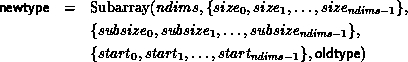Let the typemap of oldtype have the form: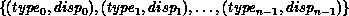where typei is a predefined MPI datatype, and let ex be the extent of oldtype. Then we define the Subarray() function recursively using the following three equations. Equation 1 defines the base step. Equation 1 defines the recursion step when order = MPI_ORDER_FORTRAN, and Equation 1 defines the recursion step when order = MPI_ORDER_C.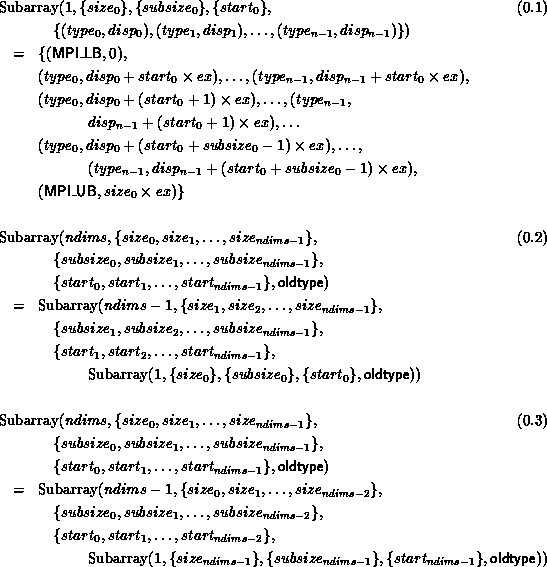For an example use of MPI_TYPE_CREATE_SUBARRAY in the context of I/O see Section Subarray Filetype Constructor .Up: New Datatype Manipulation Functions Next: Distributed Array Datatype Constructor Previous: True Extent of Datatypes